# NCERT Solutions for Class 10 Maths Chapter 3 Pair of Linear Equations in Two Variables Ex 3.5

NCERT Solutions for Class 10 Maths Chapter 3 Pair of Linear Equations in Two Variables Ex 3.5 are part of . Here are we have given Chapter 3 Pair of Linear Equations in Two Variables Class 10 NCERT Solutions Ex 3.5.

 Board CBSE Textbook NCERT Class Class 10 Subject Maths Chapter Chapter 3 Chapter Name Pair of Linear Equations in Two Variables Exercise Ex 3.5 Number of Questions Solved 4 Category NCERT Solutions

## NCERT Solutions for Class 10 Maths Chapter 3 Pair of Linear Equations in Two Variables Ex 3.5

Page No: 62

Question 1. Which of the following pairs of linear equations has unique solution, no solution or infinitely many solutions? In case there is a unique solution, find it by using cross multiplication method.
(i) x – 3y – 3 = 0 ; 3x – 9y – 2 =0
(ii) 2x + y = 5 ; 3x +2y =8
(iii) 3x – 5y = 20 ; 6x – 10y =40
(iv) x – 3y – 7 = 0 ; 3x – 3y – 15= 0

Solution: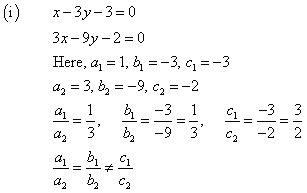Thus, the given pair of equations has no solution.Thus, the given pair of equations has unique solution.
By cross-multiplication method,Thus, the given pair of equations has infinite solutions.
The solutions can be obtained by assuming the value of x to be k, where k is any
constant. So, the ordered pairs, where k is a constant, are the solutions of
the given pair of equations.Thus, the given pair of equations has unique solution.
By cross-multiplication,Concept Insight: In order to answer such questions, remember the condition for the pair of linear equations to have unique, infinitely many or no solution relating the coefficients. Also, remember the formula used to solve by cross multiplication method. While applying the formula, be careful about the signs of the coefficients. And above all don’t forget to first write the linear equations in standard form which is ax + by + c = 0.

Question 2.
(i) For which values of a and b does the following pair of linear equations have an infinite number of solutions?
2x + 3y =7
(ab)x + (a + b)y = 3a +b –2

Solution: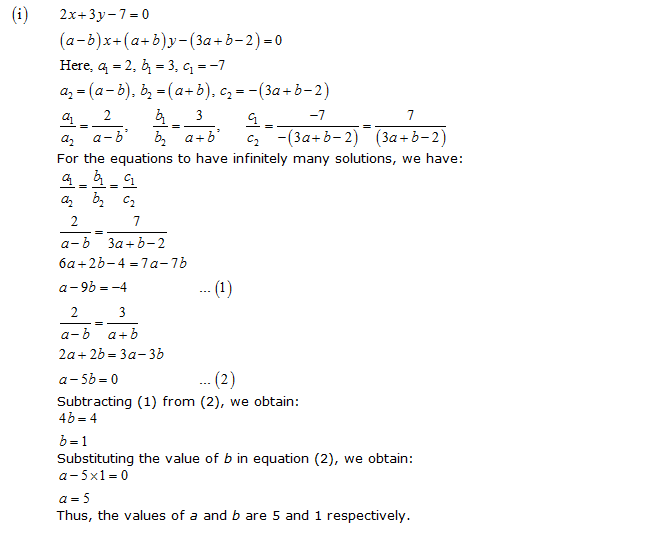(ii) For which value of k will the following pair of linear equations have no solution?
3x + y = 1
(2k –1)x + (k –1)y = 2k + 1

Solution: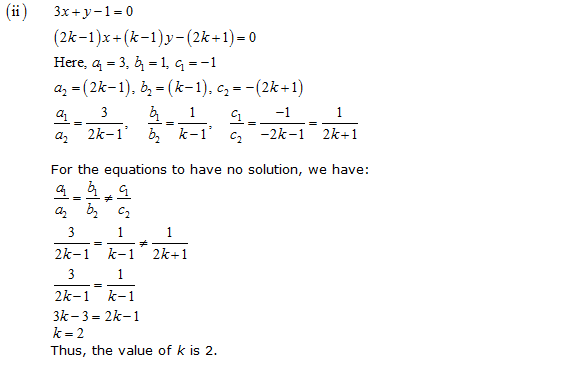Concept Insight: In order to solve such problems, firstly write the linear equations in standard form which is ax + by + c = 0.To find the value of the unknowns, the key idea is to remember the conditions for a given pair of equations to have infinite solutions and no solution.  In case of infinite solutions rule out the values which does not satisfy all the ratios. Also, be careful about the signs of the coefficients.

Question 3. Solve the following pair of linear equations by the substitution and cross-multiplication methods:
8x +5y = 9
3x +2y = 4

Solution: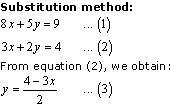Substituting this value of y in equation (1), we obtain: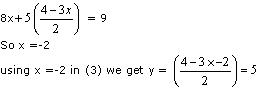∴ x = -2, y = 5
Cross-multiplication method: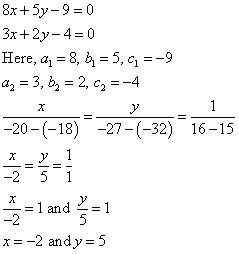Concept Insight: In order to solve the given pair of equations by cross multiplication method, remember the formula to be used and convert the system of equations to standard form. While applying the formula, be careful about the signs of the coefficients. In order to solve the given pair of equations by substitution method, substitute the value of any one of the variable from any one of the equation. Make sure you substitute the value of that variable which simplifies your calculations.
Solution will be same in both cases.

Question 4. Form the pair of linear equations in the following problems and find their solutions (if they exist) by any algebraic method:

(i) A part of monthly hostel charges is fixed and the remaining depends on the number of days one has taken food in the mess. When a student A takes food for 20 days she has to pay Rs 1000 as hostel charges whereas a student B, who takes food for 26 days, pays Rs 1180 as hostel charges. Find the fixed charges and the cost of food per day.

Solution:
Let the fixed charge of the food and the charge for food per day be x and y respectively.
According to the question,
x + 20y = 1000     …(1)
x + 26y = 1180   …(2)
Substituting this value of y in equation (1) from equation (2), we obtain
6y = 180
y = 30
Substituting this value of y in equation (1), we obtain:
x+ 20 x 30 = 1000
x = 1000 – 600
x = 400
Thus, the fixed charge of the food and the charge per day are Rs 400 and Rs 30 respectively.Concept Insight: Here, the fixed charge of the food and charge for food per day are the unknown quantities. So they are taken as variables x and y. The two equations can then be obtained by using the given conditions. You will observe that the variable x has the same coefficient in both the equations, so it will be easier to find the solution by eliminating x from both the equations. Also, one can solve the system by other methods.
(ii) A fraction becomes 1/3 when 1 is subtracted from the numerator and it becomes 1/4 when 8 is added to its denominator. Find the fraction.
Solution:
Let the fraction be x/y According to the question,
According to the question,Subtracting equation (1) from equation (2), we obtain:
x = 5
Putting the value of x in equation (1), we obtain:
15 – y = 3
y = 12
Thus the fraction is 5/12.

Concept Insight: Since the problem asks for a fraction. The numerator and denominator of the fraction need to be represented by variables. A pair of linear equations can be obtained from the given conditions. Observe that the variable y has the same coefficient in both the equations, so it will be easier to find the solution by eliminating y from both the equations.

We hope the NCERT Solutions for Class 10 Maths Chapter 3 Pair of Linear Equations in Two Variables Ex 3.5 help you. If you have any query regarding NCERT Solutions for Class 10 Maths Chapter 3 Pair of Linear Equations in Two Variables Ex 3.5, drop a comment below and we will get back to you at the earliest.